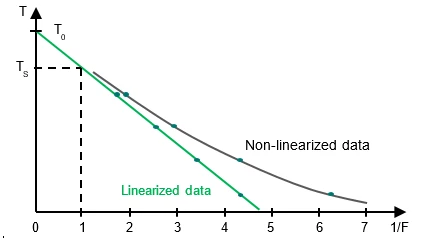Glossary

# Eutectic Purity

Eutectic Purity: What is it?

A eutectic system is a homogeneous mixture of 2 components that melts and solidifies like a pure substance. The presence of an additional component (“impurity”) causes the reduction of the Melting Temperatures and EnthalpiesThe enthalpy of fusion of a substance, also known as latent heat, is a measure of the energy input, typically heat, which is necessary to convert a substance from solid to liquid state. The melting point of a substance is the temperature at which it changes state from solid (crystalline) to liquid (isotropic melt).melting point of the main component. This effect can be highlighted with differential scanning calorimetry (DSC).## Do you have any questions?

### Suitable products for your measurement

For materials with a purity higher than 98.5 mol percent, DSC can be used to determine the amount of impurity in the mixture, or rather the purity of the main component. The method is described in ASTM E928, DIN 51004 and DIN 51007. For that, the simplified Van´t Hoff equation is used:

R: Gas constant

X2: Mole fraction of the impurity

ΔHf: Heat of fusion [J/mol]

F: Molten fraction

This equation can be rewritten as:

or as an equation of a line in a 2-dimensional x-y diagram:

y = m⋅x + b

The Ts values plotted as a function of 1 /F should yield a straight line with

• Intercept on y-axis:

T0

• Slope of the line:

In practice, the result is usually not a straight line because of pre-melting which cannot be sufficiently detected by means ofDSC. Linearization is carried out by adding a correction factor c to the total area and to each partial area:

The correction factor c has to be lower than 20% to ensure reliability of the method.Curve Ts=f(1/F) (non-linearized data) and linearized data by applying a correction factor c

The mole fraction of the impurity (X2) is obtained from the slope of the linearized curve and with knowledge of the heat of fusion of the pure substance.